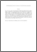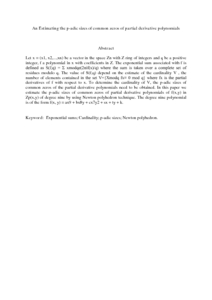# An Estimating the p-adic sizes of common zeros of partial derivative polynomials

## Citation

Sapar, Siti Hasana and Mohd Atan, Kamel Ariffin and Aminuddin, Siti Syaheera (2013) An Estimating the p-adic sizes of common zeros of partial derivative polynomials. New Trends in Mathematical Sciences, 1 (1). pp. 38-48. ISSN 2147-5520

## Abstract

Let x = (x1, x2,...,xn) be a vector in the space Zn with Z ring of integers and q be a positive integer, f a polynomial in x with coefficients in Z. The exponential sum associated with f is defined as S(f;q) = Σ xmodqe(2πif(x)/q) where the sum is taken over a complete set of residues modulo q. The value of S(f;q) depend on the estimate of the cardinality V , the number of elements contained in the set V={Xmodq fx≡ 0 mod q} where fx is the partial derivatives of f with respect to x. To determine the cardinality of V, the p-adic sizes of common zeros of the partial derivative polynomials need to be obtained. In this paper we estimate the p-adic sizes of common zeros of partial derivative polynomials of f(x,y) in Zp(x,y) of degree nine by using Newton polyhedron technique. The degree nine polynomial is of the form f(x, y) = ax9 + bx8y + cx7y2 + sx + ty + k.Preview
PDF (Abstract)
An Estimating the p.pdfView Item